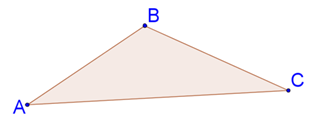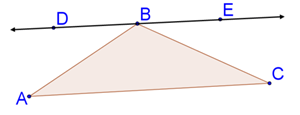# Triangle Proofs Worksheets

What Are Triangle Proofs? Recall that triangles have three sides and are a construct of three points or vertices. Where any three points can come together to form a triangle, any three lines cannot come together to form a triangle. But in order to prove this, we need some logic and theorems. Let us consider the fact that the sum of all the interior angles of a triangle is 180 degrees. We all know that this fact is true, but let us understand how this is proven to be true. Triangle Sum Theorem - Consider a triangle ABC:Take this triangle and draw a parallel line opposite to the side AC through vertex B.We know that alternate interior angles are congruent when lines are parallel. Considering this fact, we know that ∢A is congruent to ∢DBA, hence ∢A=∢DBA. Similarly, ∢C and ∢EBC are alternate interior angles showing congruence with each other, hence ∢C=∢EBC. If we observe the diagram shown above, we can see that ∢EBC, ∢ABC, ∢DBA form a straight line. Therefore, ∢EBC+ ∢ABC+ ∢DBA = 180 degrees. Hence, the statement "the sum of the measures of the interior angles of a triangle is 180 degrees" is proven. You can use this theorem to solve other geometrical problems.

• ### Basic Lesson

Guides students through the beginner skills of Triangle Proofs.

• ### Intermediate Lesson

Demonstrates how to use advanced skills to tackle Triangle Proofs problems.

• ### Independent Practice 1

A really great activity for allowing students to understand the concepts of the Triangle Proofs.

• ### Independent Practice 2

Students use Triangle Proofs in 20 assorted problems. The answers can be found below.

• ### Homework Worksheet

Students are provided with 12 problems to achieve the concepts of Triangle Proofs.

• ### Skill Quiz

This tests the students ability to understand Triangle Proofs.

Answers for all lessons and independent practice.

• ### Basic Lesson

Guides students through solving Triangles In Problems. Mathematically, similar triangles have both corresponding angles equal, while the lengths of the corresponding sides are in proportion.

• ### Intermediate Lesson

Demonstrates the concept of advanced skill while solving Triangles In Problems.

• ### Independent Practice 1

A really great activity for allowing students to understand the concepts of the Triangles In Problems.

• ### Independent Practice 2

Students use Triangles In Problems in 20 assorted problems. The answers can be found below.

• ### Homework Worksheet

Students are provided with 12 problems to achieve the concepts of Triangles In Problems.

• ### Skill Quiz

This tests the students ability to understand Triangles In Problems.

Answers for all the math worksheets and printables.

• ### Basic Lesson

Guides students through solving more of these types of problems. Two triangles are similar if and only if the corresponding sides are in proportion and the corresponding angles are congruent.

• ### Intermediate Lesson

Demonstrates the concept of advanced skill while solving these proofs. State which property is used to prove similarity.

• ### Independent Practice 1

A really great activity for allowing students to understand the concepts we have explored.

• ### Independent Practice 2

Students use the skills we have learned in 20 assorted problems. The answers can be found below.

• ### Homework Worksheet

Students are provided with 12 problems to achieve the concepts we are working on.

• ### Skill Quiz

This tests the students ability on this entire unit.